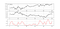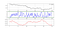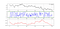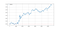## Can Correlation be Predictive? A Python Study.

Correlation is the degree of linear relationship between two or more variables. It is bounded between -1 and 1 with one being a perfectly positive correlation, -1 being a perfectly negative correlation, and 0 as an indication of no linear relationship between the variables (they relatively go in random directions). The measure is not perfect and can be biased by outliers and non-linear relationships, it does however provide quick glances to statistical properties. Two famous types of correlation exist and are commonly used:

• Spearman correlation measures the relationship between two continuous or ordinal variables. Variables may tend to change together, but not necessarily at a constant rate. It is based on the ranks of values rather than the raw data.
• Pearson correlation measures the linear relationship between two continuous variables. A relationship can be considered linear when a change in one is accompanied with a proportional change in the other.

For the first part of the article, we will stick to Pearson’s correlation measure and through its rolling values we will create an indicator to assist us in trading. For the second part of the article, we will introduce a new non-linear correlation technique called the Maximal Information Coefficient.

# Creating the AutoCorrelation Indicator — ACI

`from scipy.stats import pearsonrdef rolling_correlation(Data, first_data, second_data, lookback, where):        for i in range(len(Data)):                try:            Data[i, where] = pearsonr(Data[i - lookback + 1:i + 1, first_data], Data[i - lookback + 1:i + 1, second_data])                                except ValueError:            pass        return Data`GBPUSD in the first panel, USDCAD in the second panel, and the 50-period rolling correlation between the two. (Image by Author)

The rolling correlation measure above can help us when we want to initiate trades. For instance, imagine you find enough elements to justify a bearish position on GBPUSD and bullish elements on USDCAD. Having a strong negative correlation can help add to the trade’s conviction.

Autocorrelation is the correlation of the time series with its own lagged values. We will create the AutoCorrelation Indicator — ACI in python and then we will proceed with trading.

`from scipy.ndimage.interpolation import shiftdef adder(Data, times):        for i in range(1, times + 1):            z = np.zeros((len(Data), 1), dtype = float)        Data = np.append(Data, z, axis = 1)return Datadef auto_correlation(Data, first_data, second_data, shift_degree, lookback, where):        new_array = shift(Data[:, first_data], shift_degree, cval = 0)    new_array = np.reshape(new_array, (-1, 1))        Data = np.concatenate((Data, new_array), axis = 1)    Data = adder(Data, 20)        for i in range(len(Data)):                try:            Data[i, where] = pearsonr(Data[i - lookback + 1:i + 1, first_data], Data[i - lookback + 1:i + 1, second_data])                                except ValueError:            pass        return Data`

To understand more what these two variables are, we can provide a more formal definition:

• Lookback: This is the rolling correlation window. For example, we calculate the correlation between two datasets for the last 20 observations, then whenever we have a new observation, we include it in the lookback all while dropping the very first observation so that the window remains 20.
• Shift (shift_degree): In autocorrelation, this is the second dataset. For example, suppose we have a time series and take a shift of 1. This means that we will create a new similar time series with a lag of 1 (Yesterday’s values are put in parallel to today’s values), and then calculate the rolling correlation. In other words, it is the number of lags to account for.

The below plot shows the EURNZD values with an ACI(5, 3). This means that we are calculating the ACI with a lookback period of 5 and using an autocorrelation lag of 3.EURNZD in the first panel, the ACI(5, 3) in the second panel, and the Average True Range in the third panel. (Image by Author)

• Go long (Buy) if the ACI is lower than -0.95 with the current closing price greater than the closing price 3 periods ago. Another way to say, correlation is at an extreme low and prices are expected to continue in the same direction.
• Go long (Buy) if the ACI is greater than 0.95 with the current closing price less than the closing price 3 periods ago. Another way to say, correlation is at an extreme high and prices are expected to reverse course.
• Go short (Sell) if the ACI is greater than 0.95 with the current closing price greater than the closing price 3 periods ago. Another way to say, correlation is at an extreme high and prices are expected to reverse course.
• Go Short (Sell) if the ACI is lower than -0.95 with the current closing price less than the closing price 3 periods ago. Another way to say, correlation is at an extreme low and prices are expected to continue in the same direction.
`def signal(Data, what, buy, sell):        for i in range(len(Data)):                    if Data[i, what] < lower_barrier and Data[i - 1, what] > lower_barrier and Data[i, 3] < Data[i - 3, 3]:            Data[i, sell] = -1                    if Data[i, what] < lower_barrier and Data[i - 1, what] > lower_barrier and Data[i, 3] > Data[i - 3, 3]:            Data[i, buy] = 1                    if Data[i, what] > upper_barrier and Data[i - 1, what] < upper_barrier and Data[i, 3] < Data[i - 3, 3]:            Data[i, buy] = 1if Data[i, what] > upper_barrier and Data[i - 1, what] < upper_barrier and Data[i, 3] > Data[i - 3, 3]:            Data[i, sell] = -1`GBPNZD in the first panel, the ACI(5, 3) in the second panel, and the Average True Range in the third panel. (Image by Author)

We will once again be using an ATR-based risk management system with a cost of 0.2 pips per round trade. The back-tested data is M5 bars since November 2019 which is around 65,000 analyzed bars.Equity Curve on the GBPNZD following the Strategy. (Image by Author)

We have to do more back-tests than this to be able to incoporate the strategy into our trading framework. As the article is not about back-testing, I have not felt the need to provide more than one example. Note that generally, financial time series are not autocorrelated return-wise which makes the above results interesting. I like to use correlation to confirm my already established ideas rather than create new ones.

# A New Approach to Non-Linear Correlation: The MIC

Let us try an experiment to actually prove that the MIC can capture non-linear relationships as well. We will simulate a Sinus and Cosinus time series and then we will calculate the correlation between the two. Here’s the code to plot the below chart:

`import numpy as npimport matplotlib.pyplot as pltdata_range = np.arange(0, 30, 0.1)sine = np.sin(data_range)cosine = np.cos(data_range)plt.plot(sine, color = 'black', label = 'Sine Function')plt.plot(cosine, color = 'red', label = 'Cosine Function')plt.grid()plt.legend()`

Clearly, someone looking at the graph without knowing the functions will conclude that they are somehow correlated, whether it is the black line leading the red line or that they are both bounded by two levels. What we want to do is to calculate the MIC for these two and compare the calculation to the two other correlation measures, Spearman and Pearson. We can use the below function to do so.

`from scipy.stats import pearsonrfrom scipy.stats import spearmanrfrom minepy import MINE# Pearson Correlationprint('Correlation | Pearson: ', round(pearsonr(sine, cosine), 3))# Spearman Correlationprint('Correlation | Spearman: ', round(spearmanr(sine, cosine), 3))# MICmine = MINE(alpha = 0.6, c = 15)mine.compute_score(sine,cosine)mine.mic()print('Correlation | MIC: ', round(MIC, 3))# Output: Correlation | Pearson:  0.035# Output: Correlation | Spearman:  0.027# Output: Correlation | MIC: 0.602`

The results show the following:

• Pearson: Notice the absence of any type of correlation here due to it missing out on the non-linear association.
• Spearman: The same situation applies here with an extremely weak correlation because it does not capture non-linear relationships as indicated before.
• MIC: The measure returned a strong relationship of 0.60 between the two which is closer to reality and to what we are seeing.

The advantages of the Maximal Information Coefficient is that it is robust to outliers and it does not make any assumptions about the distribution of the variables used.

Can the MIC be used in Trading? I would like to believe that it can be. A rolling MIC measure may also be useful as an AutoCorrelation Indicator.

Note that to use the library of the Maximal Information Coefficient, we have to type the following into the Python prompt:

`pip install minepy`

# Conclusion

I always advise you to do the proper back-tests and understand any risks relating to trading. For example, the above results are not very indicative as the spread we have used is very competitive and may be considered hard to constantly obtain in the retail trading world. However, with institutional bid/ask spreads, it may be possible to lower the costs such as that a systematic medium-frequency strategy starts being profitable.

Written by

Written by

## Sofien Kaabar

#### Institutional FOREX Strategist | Trader | Data Science Enthusiast. Author of the Book of Back-tests: https://www.amazon.com/dp/B089CWQWF8